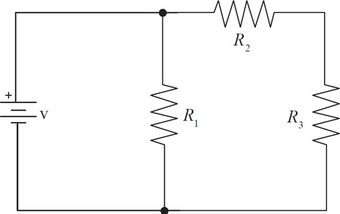# AP Physics 1 Practice Test 9

### Test Information10 questions18 minutes

1. A sound wave with frequency f travels through air at speed v. With what speed will a sound wave with frequency 4f travel through the air?

2. An object has a kinetic energy of k. If the speed of the object were doubled what would be the resulting kinetic energy?

3. A toy car and a toy truck collide. If the toy truck’s mass is double the toy car’s mass, then, compared to the acceleration of the truck, the acceleration of the car during the collision will be

4. The Gravitron is a carnival ride that looks like a large cylinder. People stand inside the cylinder against the wall as it begins to spin. Eventually, it is rotating fast enough that the floor can be removed without anyone falling. Given then the coefficient of friction between a person’s clothing and the wall is μ, the tangential speed is v, and the radius of the ride is R, what is greatest mass that a person can be to safely go on this ride?

5. In a spring-block oscillator, the maximum speed of the block is

6. A student is experimenting with a simple spring-block oscillator of spring constant k and amplitude A. The block attached to the spring has a mass of m. If the student places a small block of mass m on top of the original block, which of the following is true?

7. A pair of protective headphones reduces the sound of a jet engine from 140 dB to 110 dB. What percent of the noise is being blocked?

8. You are standing on a railroad track as a train approaches at a constant velocity. Suddenly the engineer sees you, applies the brakes, and sounds the whistle. Which of the following describes the sound of the whistle as you hear it starting from that moment?

9.Determine the total power dissipated through the circuit shown above in terms of v, R1, R2, and R3.

10.If v = 100 V, R1 = 50 Ω, R2 = 80 Ω and R3 = 120 Ω, determine the voltage across R3.